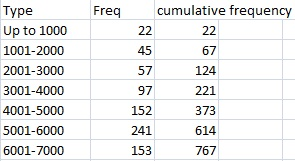# Cumulative Distribution Function CDF

Feel like "cheating" at Calculus? Check out our Practically Cheating Calculus Handbook, which gives you hundreds of easy-to-follow answers in a convenient e-book.

## What is a Cumulative Distribution Function (CDF)?

The cumulative distribution function (also called the distribution function) gives you the cumulative (additive) probability associated with a function.

For example, the CDF for a continuous random variable is the integral:It is an extension of a similar concept: a cumulative frequency table, which measures discrete counts. With a table, the frequency is the amount of times a particular number or item happens. The cumulative frequency is the total counts up to a certain number:The cumulative distribution function works in the same way, except with probabilities.

You can use the CDF to figure out probabilities above a certain value, below a certain value, or between two values. For example, if you had a CDF that showed weights of cats, you can use it to figure out:

• The probability of a cat weighing more than 11 pounds.
• The probability of a cat weighing less than 11 pounds.
• The probability of a cat weighing between 11 and 15 pounds.

In the case of the above scenario, it would be important for, say, a veterinary pharmaceutical company knowing the probability of cats weighing a certain amount in order to produce the right volume of medications that cater to certain weights.

## Cumulative Distribution Functions in Statistics

The cumulative distribution function gives the cumulative value from negative infinity up to a random variable X and is defined by the following notation:
F(x) = P(X ≤ x).
This concept is used extensively in elementary statistics, especially with z-scores. The z-table works from the idea that a score found on the table shows the probability of a random variable falling to the left of the score (some tables also show the area to some z-score to the right of the mean). The normal distribution, the basis of z-scores, is a cumulative distribution function:

## References

Abramowitz, M. and Stegun, I. A. (Eds.). “Probability Functions.” Ch. 26 in Handbook of Mathematical Functions with Formulas, Graphs, and Mathematical Tables, 9th printing. New York: Dover, pp. 925-964, 1972.
Evans, M.; Hastings, N.; and Peacock, B. Statistical Distributions, 3rd ed. New York: Wiley, pp. 6-8, 2000.
Papoulis, A. Probability, Random Variables, and Stochastic Processes, 2nd ed. New York: McGraw-Hill, pp. 92-94, 1984.
UVA: Image Retrieved April 29, 2021 from: http://www.stat.wvu.edu/srs/modules/normal/normal.html

CITE THIS AS:
Stephanie Glen. "Cumulative Distribution Function CDF" From CalculusHowTo.com: Calculus for the rest of us! https://www.calculushowto.com/cumulative-distribution-function-cdf/
---------------------------------------------------------------------------Need help with a homework or test question? With Chegg Study, you can get step-by-step solutions to your questions from an expert in the field. Your first 30 minutes with a Chegg tutor is free!